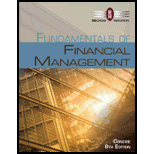# CONCEPTUAL: RETURN ON EQUITY Which of the following statements is most correct? (Hint: Work Problem 4-16 before answering 4-17, and consider the solution setup for 4-16 as you think about 4-17.) a. If a firm’s expected basic earning power (BEP) is constant for all of its assets and exceeds the interest rate on its debt, adding assets and financing them with debt will raise the firm’s expected return on common equity (ROE). b. The higher a firm’s tax rate, the lower its BEP ratio, other things held constant. c. The higher the interest rate on a firm’s debt, the lower its BEP ratio, other things held constant. d. The higher a firm’s debt ratio, the lower its BEP ratio, other things held constant. e. Statement a is false; but statements b, c, and d are true.### Fundamentals of Financial Manageme...

8th Edition
Eugene F. Brigham + 1 other
Publisher: Cengage Learning
ISBN: 9781285065137

#### Solutions

Chapter
Section### Fundamentals of Financial Manageme...

8th Edition
Eugene F. Brigham + 1 other
Publisher: Cengage Learning
ISBN: 9781285065137
Chapter 4, Problem 17P
Textbook Problem
72 views

## CONCEPTUAL: RETURN ON EQUITY Which of the following statements is most correct? (Hint: Work Problem 4-16 before answering 4-17, and consider the solution setup for 4-16 as you think about 4-17.) a. If a firm’s expected basic earning power (BEP) is constant for all of its assets and exceeds the interest rate on its debt, adding assets and financing them with debt will raise the firm’s expected return on common equity (ROE). b. The higher a firm’s tax rate, the lower its BEP ratio, other things held constant. c. The higher the interest rate on a firm’s debt, the lower its BEP ratio, other things held constant. d. The higher a firm’s debt ratio, the lower its BEP ratio, other things held constant. e. Statement a is false; but statements b, c, and d are true.

a.

Summary Introduction

To identify: The correct statement.

Basic Earnings Power (BEP):

It is the ratio of net income and total assets. Basic earning power shows the total operating of the company earned by using the assets of the company. It remains unaffected by the interest expenses and the tax rate.

The formula of basic earnings is,

Basic Earnings Power=Net IncomeTotal Assets

### Explanation of Solution

• Basic earning power is determined by dividing EBIT by total assets. It is influenced by the tax rate and interest expenses.
• So, BEP remains constant for all its assets even if the rate changes...

b.

Summary Introduction

To identify: The correct statement.

Basic Earnings Power (BEP):

It is the ratio of net income and total assets. Basic earning power shows the total operating of the company earned by using the assets of the company. It remains unaffected by the interest expenses and the tax rate.

The formula of basic earnings is,

Basic Earnings Power=Net IncomeTotal Assets

c.

Summary Introduction

To identify: The correct statement.

Basic Earnings Power (BEP):

It is the ratio of net income and total assets. Basic earning power shows the total operating of the company earned by using the assets of the company. It remains unaffected by the interest expenses and the tax rate.

The formula of basic earnings is,

Basic Earnings Power=Net IncomeTotal Assets

d.

Summary Introduction

To identify: The correct statement.

Basic Earnings Power (BEP):

It is the ratio of net income and total assets. Basic earning power shows the total operating of the company earned by using the assets of the company. It remains unaffected by the interest expenses and the tax rate.

The formula of basic earnings is,

Basic Earnings Power=Net IncomeTotal Assets

### Still sussing out bartleby?

Check out a sample textbook solution.

See a sample solution

#### The Solution to Your Study Problems

Bartleby provides explanations to thousands of textbook problems written by our experts, many with advanced degrees!

Get Started